Next: Exercises Up: Two-Dimensional Incompressible Inviscid Flow Previous: Inviscid Flow Over a

# Two-Dimensional Jets

Consider a two-dimensional jet of width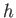and uniform speed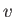that impinges on a rigid plate, at an angle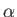to the normal to the plate, and divides into two streams, running along the plate, whose widths are ultimately uniform, and equal toand. (See Figure 5.14.) We expect the pressure well upstream of the plate to be atmospheric all the way across the jet, because the jet is not yet decelerating. Likewise, we expect the pressure well downstream of the strike point to be atmospheric all the way across the two streams that run along the plate, because the streams are no longer accelerating (and, hence, their widths are constant). It immediately follows from Bernoulli's theorem that the asymptotic flow speed in the two streams is, as indicated in Figure 5.14.Fluid continuity demands that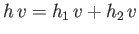, or(5.134)

Moreover, because the plate can only exert a normal force on the fluid, it follows that the parallel (to the plate) momentum flux of the incident jet must equal the net parallel momentum flux of the two streams. In other words,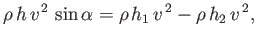(5.135)

or(5.136)

Equations (5.134) and (5.136) can be combined to give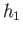(5.137)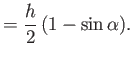(5.138)Let us apply the Euler momentum theorem (see Section 4.4) to the flux tube shown in Figure 5.14, treating the plate as one of the walls of the tube. By symmetry, the net force (per unit width perpendicular to the page) exerted across the walls and ends of the tube is the resultant of the three forces shown in Figure 5.15. It is easily demonstrated that this net force,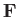, is normal to the plate, as shown in the figure, and of magnitude(5.139)

Obviously, the force in question corresponds to the force exerted by the plate on the fluid. An equal and opposite force is exerted by the fluid on the plate. (Note that there is no net pressure force on the flux tube because it is entirely surrounded by fluid at the same pressure: i.e., atmospheric pressure.) The line of action of the resultant force passes through the so-called center of pressure,. This is displaced slightly from the point,, where the middle of the jet strikes the plate. (See Figure 5.15.) Let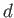be the distance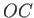. We can calculateby demanding that the net moment of the three forces shown in Figure 5.15 about pointbe zero. In other words,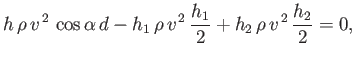(5.140)

which yields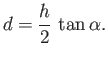(5.141)Next: Exercises Up: Two-Dimensional Incompressible Inviscid Flow Previous: Inviscid Flow Over a
Richard Fitzpatrick 2016-03-31